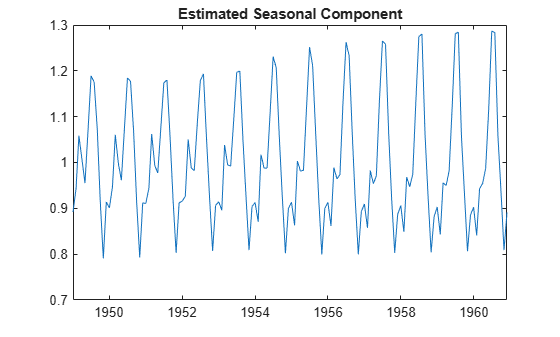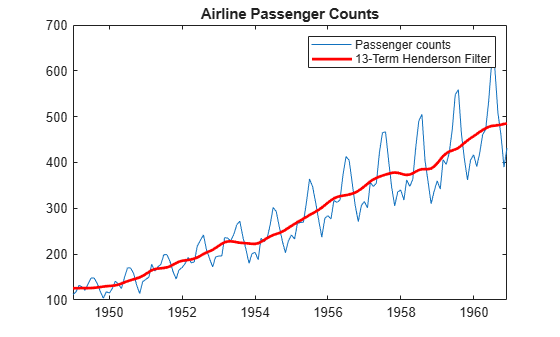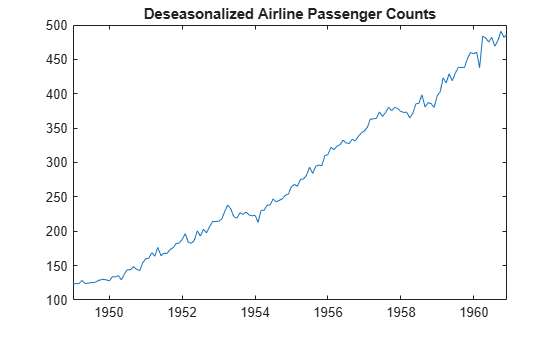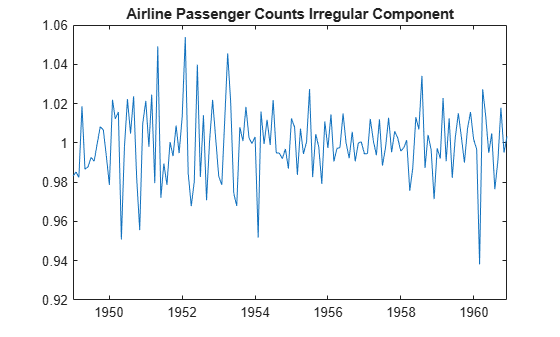# Seasonal Adjustment Using S(n,m) Seasonal Filters

This example shows how to apply ${S}_{n×m}$ seasonal filters to deseasonalize a time series (using a multiplicative decomposition). The time series is monthly international airline passenger counts from 1949 to 1960.

Load the airline data set.

```load Data_Airline y = DataTimeTable.PSSG; T = length(y); figure plot(DataTimeTable.Time,y) title('Airline Passenger Counts') hold on```The data shows an upward linear trend and a seasonal component with periodicity 12.

### Detrend Data Using 13-term Moving Average

Before estimating the seasonal component, estimate and remove the linear trend. Apply a 13-term symmetric moving average, repeating the first and last observations six times to prevent data loss. Use weight 1/24 for the first and last terms in the moving average, and weight 1/12 for all interior terms.

Divide the original series by the smoothed series to detrend the data. Add the moving average trend estimate to the observed time series plot.

```sW13 = [1/24;repmat(1/12,11,1);1/24]; yS = conv(y,sW13,'same'); yS(1:6) = yS(7); yS(T-5:T) = yS(T-6); xt = y./yS; plot(DataTable.Time,yS,'r','LineWidth',2) legend(["Passenger counts" "13-Term Moving Average"]) hold off```### Create Seasonal Indices

Create a cell array, `sidx`, to store the indices corresponding to each period. The data is monthly, with periodicity 12, so the first element of `sidx` is a vector with elements 1, 13, 25,...,133 (corresponding to January observations). The second element of `sidx` is a vector with elements 2, 14, 16,...,134 (corresponding to February observations). This is repeated for all 12 months.

```s = 12; sidx = cell(s,1); % Preallocation for i = 1:s sidx{i,1} = i:s:T; end sidx{1:2}```
```ans = 1×12 1 13 25 37 49 61 73 85 97 109 121 133 ```
```ans = 1×12 2 14 26 38 50 62 74 86 98 110 122 134 ```

Using a cell array to store the indices allows for the possibility that each period does not occur the same number of times within the span of the observed series.

### Apply S(3,3) Filter

Apply a 5-term ${S}_{3×3}$ seasonal moving average to the detrended series `xt`. That is, apply a moving average to the January values (at indices 1, 13, 25,...,133), and then apply a moving average to the February series (at indices 2, 14, 26,...,134), and so on for the remaining months.

Use asymmetric weights at the ends of the moving average (using `conv2`). Put the smoothed values back into a single vector.

To center the seasonal component around one, estimate, and then divide by, a 13-term moving average of the estimated seasonal component.

```% S3x3 seasonal filter % Symmetric weights sW3 = [1/9;2/9;1/3;2/9;1/9]; % Asymmetric weights for end of series aW3 = [.259 .407;.37 .407;.259 .185;.111 0]; % Apply filter to each month shat = NaN*y; for i = 1:s ns = length(sidx{i}); first = 1:4; last = ns - 3:ns; dat = xt(sidx{i}); sd = conv(dat,sW3,'same'); sd(1:2) = conv2(dat(first),1,rot90(aW3,2),'valid'); sd(ns -1:ns) = conv2(dat(last),1,aW3,'valid'); shat(sidx{i}) = sd; end % 13-term moving average of filtered series sW13 = [1/24;repmat(1/12,11,1);1/24]; sb = conv(shat,sW13,'same'); sb(1:6) = sb(s+1:s+6); sb(T-5:T) = sb(T-s-5:T-s); % Center to get final estimate s33 = shat./sb; figure plot(DataTimeTable.Time,s33) title('Estimated Seasonal Component')```Notice that the seasonal level changes over the range of the data. This illustrates the difference between an ${S}_{n×m}$ seasonal filter and a stable seasonal filter. A stable seasonal filter assumes that the seasonal level is constant over the range of the data.

### Apply 13-term Henderson Filter

To get an improved estimate of the trend component, apply a 13-term Henderson filter to the seasonally adjusted series. The necessary symmetric and asymmetric weights are provided in the following code.

```% Deseasonalize series dt = y./s33; % Henderson filter weights sWH = [-0.019;-0.028;0;.066;.147;.214; .24;.214;.147;.066;0;-0.028;-0.019]; % Asymmetric weights for end of series aWH = [-.034 -.017 .045 .148 .279 .421; -.005 .051 .130 .215 .292 .353; .061 .135 .201 .241 .254 .244; .144 .205 .230 .216 .174 .120; .211 .233 .208 .149 .080 .012; .238 .210 .144 .068 .002 -.058; .213 .146 .066 .003 -.039 -.092; .147 .066 .004 -.025 -.042 0 ; .066 .003 -.020 -.016 0 0 ; .001 -.022 -.008 0 0 0 ; -.026 -.011 0 0 0 0 ; -.016 0 0 0 0 0 ]; % Apply 13-term Henderson filter first = 1:12; last = T-11:T; h13 = conv(dt,sWH,'same'); h13(T-5:end) = conv2(dt(last),1,aWH,'valid'); h13(1:6) = conv2(dt(first),1,rot90(aWH,2),'valid'); % New detrended series xt = y./h13; figure plot(DataTimeTable.Time,y) hold on plot(DataTimeTable.Time,h13,'r','LineWidth',2); legend(["Passenger counts" "13-Term Henderson Filter"]) title('Airline Passenger Counts') hold off```### Apply S(3,5) Seasonal Filter

To get 6. an improved estimate of the seasonal component, apply a 7-term ${S}_{3×5}$ seasonal moving average to the newly detrended series. The symmetric and asymmetric weights are provided in the following code. Center the seasonal estimate to fluctuate around 1.

Deseasonalize the original series by dividing it by the centered seasonal estimate.

```% S3x5 seasonal filter % Symmetric weights sW5 = [1/15;2/15;repmat(1/5,3,1);2/15;1/15]; % Asymmetric weights for end of series aW5 = [.150 .250 .293; .217 .250 .283; .217 .250 .283; .217 .183 .150; .133 .067 0; .067 0 0]; % Apply filter to each month shat = NaN*y; for i = 1:s ns = length(sidx{i}); first = 1:6; last = ns-5:ns; dat = xt(sidx{i}); sd = conv(dat,sW5,'same'); sd(1:3) = conv2(dat(first),1,rot90(aW5,2),'valid'); sd(ns-2:ns) = conv2(dat(last),1,aW5,'valid'); shat(sidx{i}) = sd; end % 13-term moving average of filtered series sW13 = [1/24;repmat(1/12,11,1);1/24]; sb = conv(shat,sW13,'same'); sb(1:6) = sb(s+1:s+6); sb(T-5:T) = sb(T-s-5:T-s); % Center to get final estimate s35 = shat./sb; % Deseasonalized series dt = y./s35; figure plot(DataTimeTable.Time,dt) title('Deseasonalized Airline Passenger Counts')```The deseasonalized series consists of the long-term trend and irregular components. With the seasonal component removed, it is easier to see turning points in the trend.

### Plot the components and the original series.

Compare the original series to a series reconstructed using the component estimates.

```figure plot(DataTimeTable.Time,y,'Color',[.85,.85,.85],'LineWidth',4) hold on plot(DataTimeTable.Time,h13,'r','LineWidth',2) plot(DataTimeTable.Time,h13.*s35,'k--','LineWidth',1.5) legend(["Passenger counts" "13-Term Henderson Filter" ... "Trend and Seasonal Components"]) hold off title('Airline Passenger Counts')```### Estimate Irregular Component

Detrend and deseasonalize the original series. Plot the remaining estimate of the irregular component.

```Irr = dt./h13; figure plot(DataTimeTable.Time,Irr) title('Airline Passenger Counts Irregular Component')```You can optionally model the detrended and deseasonalized series using a stationary stochastic process model.